We use cookies and other tracking technologies to improve your browsing experience on our site, analyze site traffic, and understand where our audience is coming from. To find out more, please read our privacy policy.

By choosing 'I Accept', you consent to our use of cookies and other tracking technologies.

We use cookies and other tracking technologies to improve your browsing experience on our site, analyze site traffic, and understand where our audience is coming from. To find out more, please read our privacy policy.

By choosing 'I Accept', you consent to our use of cookies and other tracking technologies. Less

We use cookies and other tracking technologies... More

# Login or registerto boost this post!

Show some love to the author of this blog by giving their post some rocket fuel 🚀.

# Login to see the application

Engineers who find a new job through Golang Works average a 15% increase in salary 🚀# Top 10 Machine Learning Algorithms for Beginners

Reena Shaw 15 April, 2019 | 14 min read

## I. Introduction

The study of ML algorithms has gained immense traction post the Harvard Business Review article terming a ‘Data Scientist’ as the ‘Sexiest job of the 21st century’. So, for those starting out in the field of ML, we decided to do a reboot of our immensely popular Gold blog The 10 Algorithms Machine Learning Engineers need to know - albeit this post is targetted towards beginners.

ML algorithms are those that can learn from data and improve from experience, without human intervention. Learning tasks may include learning the function that maps the input to the output, learning the hidden structure in unlabeled data; or ‘instance-based learning’, where a class label is produced for a new instance by comparing the new instance (row) to instances from the training data, which were stored in memory. ‘Instance-based learning’ does not create an abstraction from specific instances.

## II. Types of ML algorithms

There are 3 types of ML algorithms:

1. Supervised learning:

Supervised learning can be explained as follows: use labeled training data to learn the mapping function from the input variables (X) to the output variable (Y).

Y = f (X)

Supervised learning problems can be of two types:

a. Classification: To predict the outcome of a given sample where the output variable is in the form of categories. Examples include labels such as male and female, sick and healthy.

b. Regression: To predict the outcome of a given sample where the output variable is in the form of real values. Examples include real-valued labels denoting the amount of rainfall, the height of a person.

The 1st 5 algorithms that we cover in this blog– Linear Regression, Logistic Regression, CART, Naïve Bayes, KNN are examples of supervised learning.

Ensembling is a type of supervised learning. It means combining the predictions of multiple different weak ML models to predict on a new sample. Algorithms 9-10 that we cover– Bagging with Random Forests, Boosting with XGBoost are examples of ensemble techniques.

2. Unsupervised learning:

Unsupervised learning problems possess only the input variables (X) but no corresponding output variables. It uses unlabeled training data to model the underlying structure of the data.

Unsupervised learning problems can be of two types:

a. Association: To discover the probability of the co-occurrence of items in a collection. It is extensively used in market-basket analysis. Example: If a customer purchases bread, he is 80% likely to also purchase eggs.

b. Clustering: To group samples such that objects within the same cluster are more similar to each other than to the objects from another cluster.

c. Dimensionality Reduction: True to its name, Dimensionality Reduction means reducing the number of variables of a dataset while ensuring that important information is still conveyed. Dimensionality Reduction can be done using Feature Extraction methods and Feature Selection methods. Feature Selection selects a subset of the original variables. Feature Extraction performs data transformation from a high-dimensional space to a low-dimensional space. Example: PCA algorithm is a Feature Extraction approach.

Algorithms 6-8 that we cover here - Apriori, K-means, PCA are examples of unsupervised learning.

3. Reinforcement learning:

Reinforcement learning is a type of machine learning algorithm that allows the agent to decide the best next action based on its current state, by learning behaviours that will maximize the reward.

Reinforcement algorithms usually learn optimal actions through trial and error. They are typically used in robotics – where a robot can learn to avoid collisions by receiving negative feedback after bumping into obstacles, and in video games – where trial and error reveals specific movements that can shoot up a player’s rewards. The agent can then use these rewards to understand the optimal state of game play and choose the next action.

## III. Quantifying the popularity of ML algorithms

Survey papers such as these have quantified the 10 most popular data mining algorithms. However, such lists are subjective and as in the case of the quoted paper, the sample size of the polled participants is very narrow and consists of advanced practitioners of data mining. The persons polled were the winners of the ACM KDD Innovation Award, the IEEE ICDM Research Contributions Award; the Program Committee members of the KDD-06, ICDM’06 and SDM’06; and the 145 attendees of the ICDM’06.

The Top 10 algorithms in this blog are meant for beginners and are primarily those that I learnt from the ‘Data Warehousing and Mining’ (DWM) course during my Bachelor’s degree in Computer Engineering at the University of Mumbai. The DWM course is a great introduction to the field of ML algorithms. I have especially included the last 2 algorithms (ensemble methods) based on their prevalence to win Kaggle competitions](http://www.datasciencecentral.com/profiles/blogs/want-to-win-at-kaggle-pay-attention-to-your-ensembles). Hope you enjoy the article!

## IV. Supervised learning algorithms

1. Linear Regression

In ML, we have a set of input variables (x) that are used to determine the output variable (y). A relationship exists between the input variables and the output variable. The goal of ML is to quantify this relationship.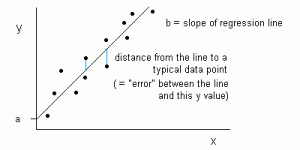Figure 1: Linear Regression is represented as a line in the form of y = a + bx. Source

In Linear Regression, the relationship between the input variables (x) and output variable (y) is expressed as an equation of the form y = a + bx. Thus, the goal of linear regression is to find out the values of coefficients a and b. Here, a is the intercept and b is the slope of the line.

Figure 1 shows the plotted x and y values for a dataset. The goal is to fit a line that is nearest to most of the points. This would reduce the distance (‘error’) between the y value of a data point and the line.

2. Logistic Regression

Linear regression predictions are continuous values (rainfall in cm),logistic regression predictions are discrete values (whether a student passed/failed) after applying a transformation function.

Logistic regression is best suited for binary classification (datasets where y = 0 or 1, where 1 denotes the default class. Example: In predicting whether an event will occur or not, the event that it occurs is classified as 1. In predicting whether a person will be sick or not, the sick instances are denoted as 1). It is named after the transformation function used in it, called the logistic function h(x)= 1/ (1 + ex), which is an S-shaped curve.

In logistic regression, the output is in the form of probabilities of the default class (unlike linear regression, where the output is directly produced). As it is a probability, the output lies in the range of 0-1. The output (y-value) is generated by log transforming the x-value, using the logistic function h(x)= 1/ (1 + e^ -x) . A threshold is then applied to force this probability into a binary classification.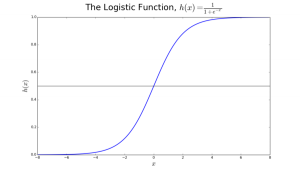Figure 2: Logistic Regression to determine if a tumour is malignant or benign. Classified as malignant if the probability h(x)>= 0.5. Source

In Figure 2, to determine whether a tumour is malignant or not, the default variable is y=1 (tumour= malignant) ; the x variable could be a measurement of the tumour, such as the size of the tumour. As shown in the figure, the logistic function transforms the x-value of the various instances of the dataset, into the range of 0 to 1. If the probability crosses the threshold of 0.5 (shown by the horizontal line), the tumour is classified as malignant.

The logistic regression equation P(x) = e ^ (b0 +b1x) / (1 + e(b0 + b1x)) can be transformed into ln(p(x) / 1-p(x)) = b0 + b1*x.

The goal of logistic regression is to use the training data to find the values of coefficients b0 and b1 such that it will minimize the error between the predicted outcome and the actual outcome. These coefficients are estimated using the technique of Maximum Likelihood Estimation.

3. CART

Classification and Regression Trees (CART) is an implementation of Decision Trees, among others such as ID3, C4.5.

The non-terminal nodes are the root node and the internal node. The terminal nodes are the leaf nodes. Each non-terminal node represents a single input variable (x) and a splitting point on that variable; the leaf nodes represent the output variable (y). The model is used as follows to make predictions: walk the splits of the tree to arrive at a leaf node and output the value present at the leaf node.

The decision tree in Figure3 classifies whether a person will buy a sports car or a minivan depending on their age and marital status. If the person is over 30 years and is not married, we walk the tree as follows : ‘over 30 years?’ -> yes -> ’married?’ -> no. Hence, the model outputs a sportscar.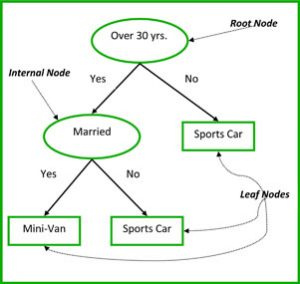Figure 3: Parts of a decision tree. Source

4. Naïve Bayes

To calculate the probability that an event will occur, given that another event has already occurred, we use Bayes’ Theorem. To calculate the probability of an outcome given the value of some variable, that is, to calculate the probability of a hypothesis(h) being true, given our prior knowledge(d), we use Bayes’ Theorem as follows:

P(h|d)= (P(d|h) * P(h)) / P(d)

where:

• P(h|d) = Posterior probability. The probability of hypothesis h being true, given the data d, where P(h|d)= P(d1| h) P(d2| h)....P(dn| h) P(d)
• P(d|h) = Likelihood. The probability of data d given that the hypothesis h was true.
• P(h) = Class prior probability. The probability of hypothesis h being true (irrespective of the data)
• P(d) = Predictor prior probability. Probability of the data (irrespective of the hypothesis)

• This algorithm is called ‘naive’ because it assumes that all the variables are independent of each other, which is a naive assumption to make in real-world examples.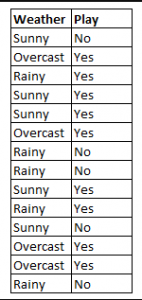Figure 4: Using Naive Bayes to predict the status of ‘play’ using the variable ‘weather’.

Using Figure 4 as an example, what is the outcome if weather=’sunny’?

To determine the outcome play= ‘yes’ or ‘no’ given the value of variable weather=’sunny’, calculate P(yes|sunny) and P(no|sunny) and choose the outcome with higher probability.

->P(yes|sunny)= (P(sunny|yes) * P(yes)) / P(sunny) = (3/9 * 9/14 ) / (5/14) = 0.60

-> P(no|sunny)= (P(sunny|no) * P(no)) / P(sunny) = (2/5 * 5/14 ) / (5/14) = 0.40

Thus, if the weather =’sunny’, the outcome is play= ‘yes’.

5. KNN

The k-nearest neighbours algorithm uses the entire dataset as the training set, rather than splitting the dataset into a trainingset and testset.

When an outcome is required for a new data instance, the KNN algorithm goes through the entire dataset to find the k-nearest instances to the new instance, or the k number of instances most similar to the new record, and then outputs the mean of the outcomes (for a regression problem) or the mode (most frequent class) for a classification problem. The value of k is user-specified.

The similarity between instances is calculated using measures such as Euclidean distance and Hamming distance.

## V. Unsupervised learning algorithms:

6. Apriori

The Apriori algorithm is used in a transactional database to mine frequent itemsets and then generate association rules. It is popularly used in market basket analysis, where one checks for combinations of products that frequently co-occur in the database. In general, we write the association rule for ‘if a person purchases item X, then he purchases item Y’ as : X -> Y.

Example: if a person purchases milk and sugar, then he is likely to purchase coffee powder. This could be written in the form of an association rule as: {milk,sugar} -> coffee powder. Association rules are generated after crossing the threshold for support and confidence.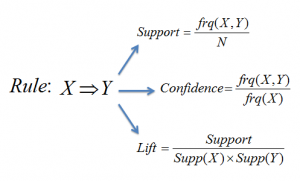Figure 5: Formulae for support, confidence and lift for the association rule X->Y. Source

The Support measure helps prune the number of candidate itemsets to be considered during frequent itemset generation. This support measure is guided by the Apriori principle. The Apriori principle states that if an itemset is frequent, then all of its subsets must also be frequent.

7. K-means

K-means is an iterative algorithm that groups similar data into clusters.It calculates the centroids of k clusters and assigns a data point to that cluster having least distance between its centroid and the data point.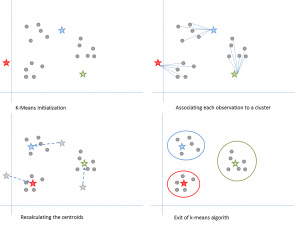Figure 6: Steps of the K-means algorithm. Source

Step 1: k-means initialization:

a) Choose a value of k. Here, let us take k=3. b) Randomly assign each data point to any of the 3 clusters. c) Compute cluster centroid for each of the clusters. The red, blue and green stars denote the centroids for each of the 3 clusters.

Step 2: Associating each observation to a cluster:

Reassign each point to the closest cluster centroid. Here, the upper 5 points got assigned to the cluster with the blue colour centroid. Follow the same procedure to assign points to the clusters containing the red and green colour centroid.

Step 3: Recalculating the centroids:

Calculate the centroids for the new clusters. The old centroids are shown by gray stars while the new centroids are the red, green and blue stars.

Step 4: Iterate, then exit if unchanged.

Repeat steps 2-3 until there is no switching of points from one cluster to another. Once there is no switching for 2 consecutive steps, exit the k-means algorithm.

8. PCA

Principal Component Analysis (PCA) is used to make data easy to explore and visualize by reducing the number of variables. This is done by capturing the maximum variance in the data into a new co-ordinate system with axes called ‘principal components’. Each component is a linear combination of the original variables and is orthogonal to one another. Orthogonality between components indicates that the correlation between these components is zero.

The first principal component captures the direction of the maximum variability in the data. The second principal component captures the remaining variance in the data but has variables uncorrelated with the first component. Similarly, all successive principal components (PC3, PC4 and so on) capture the remaining variance while being uncorrelated with the previous component.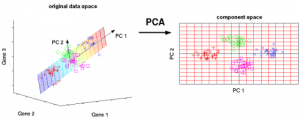Figure 7: The 3 original variables (genes) are reduced to 2 new variables termed principal components (PC's). Source

## VI. Ensemble learning techniques:

Ensembling means combining the results of multiple learners (classifiers) for improved results, by voting or averaging. Voting is used during classification and averaging is used during regression. The idea is that ensembles of learners perform better than single learners.

There are 3 types of ensembling algorithms: Bagging, Boosting and Stacking. We are not going to cover ‘stacking’ here, but if you’d like a detailed explanation of it, let me know in the comments section below, and I can write a separate blog on it.

9. Bagging with Random Forests

Random Forest (multiple learners) is an improvement over bagged decision trees (a single learner).

Bagging: The first step in bagging is to create multiple models with datasets created using the Bootstrap Sampling method. In Bootstrap Sampling, each generated trainingset is composed of random subsamples from the original dataset. Each of these trainingsets is of the same size as the original dataset, but some records repeat multiple times and some records do not appear at all. Then, the entire original dataset is used as the testset. Thus, if the size of the original dataset is N, then the size of each generated trainingset is also N, with the number of unique records being about (2N/3); the size of the testset is also N.

The second step in bagging is to create multiple models by using the same algorithm on the different generated trainingsets. In this case, let us discuss Random Forest. Unlike a decision tree, where each node is split on the best feature that minimizes error, in random forests, we choose a random selection of features for constructing the best split. The reason for randomness is: even with bagging, when decision trees choose a best feature to split on, they end up with similar structure and correlated predictions. But bagging after splitting on a random subset of features means less correlation among predictions from subtrees.

The number of features to be searched at each split point is specified as a parameter to the random forest algorithm.

Thus, in bagging with Random Forest, each tree is constructed using a random sample of records and each split is constructed using a random sample of predictors.

a) Bagging is a parallel ensemble because each model is built independently. On the other hand, boosting is a sequential ensemble where each model is built based on correcting the misclassifications of the previous model.

b) Bagging mostly involves ‘simple voting’, where each classifier votes to obtain a final outcome– one that is determined by the majority of the parallel models; boosting involves ‘weighted voting’, where each classifier votes to obtain a final outcome which is determined by the majority– but the sequential models were built by assigning greater weights to misclassified instances of the previous models.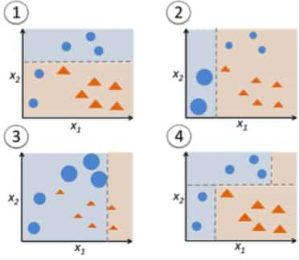Figure 9: Adaboost for a decision tree. Source

In Figure 9, steps 1, 2, 3 involve a weak learner called a decision stump (a 1-level decision tree making a prediction based on the value of only 1 input feature; a decision tree with its root immediately connected to its leaves). The process of constructing weak learners continues until a user-defined number of weak learners has been constructed or until there is no further improvement while training. Step 4 combines the 3 decision stumps of the previous models (and thus has 3 splitting rules in the decision tree).

Step 1: Start with 1 decision tree stump to make a decision on 1 input variable:

The size of the data points show that we have applied equal weights to classify them as a circle or triangle. The decision stump has generated a horizontal line in the top half to classify these points. We can see that there are 2 circles incorrectly predicted as triangles. Hence, we will assign higher weights to these 2 circles and apply another decision stump.

Step 2: Move to another decision tree stump to make a decision on another input variable:

We observe that the size of the 2 misclassified circles from the previous step is larger than the remaining points. Now, the 2nd decision stump will try to predict these 2 circles correctly.

As a result of assigning higher weights, these 2 circles have been correctly classified by the vertical line on the left. But this has now resulted in misclassifying the 3 circles at the top. Hence, we will assign higher weights to these 3 circles at the top and apply another decision stump.

Step 3: Train another decision tree stump to make a decision on another input variable.

The 3 misclassified circles from the previous step are larger than the rest of the data points. Now, a vertical line to the right has been generated to classify the circles and triangles.

Step 4: Combine the decision stumps:

We have combined the separators from the 3 previous models and observe that the complex rule from this model classifies data points correctly as compared to any of the individual weak learners.

## VII. Conclusion:

To recap, we have learnt:

• 5 supervised learning techniques- Linear Regression, Logistic Regression, CART, Naïve Bayes, KNN.
• 3 unsupervised learning techniques- Apriori, K-means, PCA.
• 2 ensembling techniques- Bagging with Random Forests, Boosting with XGBoost.

• In her next blog, we will learn about a technique that has become a rage at Kaggle competitions - the XGBoost algorithm.

Reena Shaw is a lover of all things data, spicy food and Alfred Hitchcock. She recently graduated with a Master's in Computer Science from Queen's University with her Thesis in Deep Learning, and resides in Toronto. Contact her on: Linkedin | 14rsm5@queensu.ca | @ReenaShawLegacy

Originally published on www.kdnuggets.com

## Related Issues

cosmos / gaia
• Started
• 0
• 7
• Intermediate
• Go
cosmos / gaia
• Started
• 0
• 3
• Intermediate
• Go
cosmos / ibc
• Started
• 0
• 2
• Intermediate
• TeX
cosmos / ibc
• Open
• 0
• 0
• Intermediate
• TeX

### Get hired!

#### Sign up now and apply for roles at companies that interest you.Engineers who find a new job through Golang Works average a 15% increase in salary.